# Generate C Code from Symbolic Expressions Using the MATLAB Coder App

This example shows how to use the MATLAB® Coder™ app to generate a static C library from symbolic expressions. First, you work with symbolic expressions in Symbolic Math Toolbox™, and convert the symbolic expressions into a deployable MATLAB function using `matlabFunction`. Next, you generate C code from the MATLAB function. The generated C code accepts inputs that have a fixed, preassigned size, but you can also specify variable-size inputs during code generation.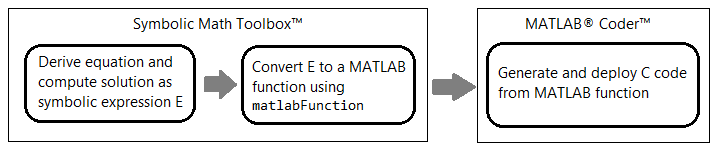This example follows the steps described in Generate C Code by Using the MATLAB Coder App (MATLAB Coder), but updates the steps to generate a MATLAB function from a symbolic expression. Alternatively, you can generate C code from a MATLAB function at the MATLAB command line by using the `codegen` (MATLAB Coder) command. For a tutorial on this workflow, see Generate C Code at the Command Line (MATLAB Coder).

Note that the MATLAB Coder app is not supported in MATLAB Online™. To generate C/C++ code in MATLAB Online, use the `codegen` (MATLAB Coder) command.

### Generate Deployable MATLAB Function from Symbolic Expression

This example solves for the eigenvalues of the model Hamiltonian:

`$\mathit{H}=\left(\begin{array}{cc}{\left(q-1\right)}^{2}-\frac{\delta }{2}& \Omega \\ \Omega & \frac{\delta }{2}+{\left(q+1\right)}^{2}\end{array}\right),$`

where $q$, $\Omega$, and $\delta$ are the parameters of the Hamiltonian.

Create the symbolic variables `q`, `Omega`, and `delta` to represent the parameters of the Hamiltonian. Create a symbolic matrix for the Hamiltonian.

```syms q Omega delta H = [(q-1)^2 - delta/2, Omega; Omega, (q+1)^2 + delta/2]```
```H =  $\left(\begin{array}{cc}{\left(q-1\right)}^{2}-\frac{\delta }{2}& \Omega \\ \Omega & \frac{\delta }{2}+{\left(q+1\right)}^{2}\end{array}\right)$```

Find the two eigenvalues of the Hamiltonian.

`E = eig(H)`
```E =  $\left(\begin{array}{c}{q}^{2}-\frac{\sqrt{4 {\Omega }^{2}+{\delta }^{2}+8 \delta q+16 {q}^{2}}}{2}+1\\ {q}^{2}+\frac{\sqrt{4 {\Omega }^{2}+{\delta }^{2}+8 \delta q+16 {q}^{2}}}{2}+1\end{array}\right)$```

Next, convert the two eigenvalues `E(1)` and `E(2)` to a MATLAB function file by using `matlabFunction`. Write the resulting function, which returns two elements `E1` and `E2`, to the file `myEigenvalues.m`. Specify the order of input arguments as `[q Omega delta]`.

```matlabFunction(E(1),E(2),'File','myEigenvalues', ... 'Vars',[q Omega delta],'Outputs',{'E1','E2'});```

The converted function in the file `myEigenvalues.m` can be used without Symbolic Math Toolbox. The MATLAB file `myEigenvalues.m` contains the function `myEigenvalues` that implements the core algorithm in this example. The function takes `q`, `Omega`, and `delta` as inputs, all of which must be either the same size or a scalar. It then calculates the two eigenvalues as a function of these inputs.

`type myEigenvalues`
```function [E1,E2] = myEigenvalues(q,Omega,delta) %myEigenvalues % [E1,E2] = myEigenvalues(Q,Omega,DELTA) % This function was generated by the Symbolic Math Toolbox version 9.0. % 01-Sep-2021 16:44:40 t2 = Omega.^2; t3 = delta.^2; t4 = q.^2; t6 = delta.*q.*8.0; t5 = t2.*4.0; t7 = t4.*1.6e+1; t8 = t3+t5+t6+t7; t9 = sqrt(t8); t10 = t9./2.0; E1 = t4-t10+1.0; if nargout > 1 E2 = t4+t10+1.0; end ```

### Run MATLAB Test Script

To calculate the eigenvalues for a set of inputs, create and run the test script `myTest.m` in MATLAB. The test script specifies the inputs with the following sizes:

• `qGrid` is a `128`-by-`256` matrix that represents points in the two-dimensional ($q$,$\Omega$) space.

• `OmegaGrid` is a `128`-by-`256` matrix that represents points in the two-dimensional ($q$,$\Omega$) space.

• `delta` is a scalar.

The script then calls the function `myEigenvalues.m` to compute the eigenvalues. The output displays a plot of the eigenvalues for these input values. Below is the content of the script `myTest.m`.

```q = linspace(-2,2,256); Omega = linspace(0,2,128); delta = 1; [qGrid,OmegaGrid] = meshgrid(q,Omega); [E1,E2] = myEigenvalues(qGrid,OmegaGrid,delta); surf(q,Omega,E1) hold on; surf(q,Omega,E2) shading interp```### Generate C Code from MATLAB Function

To make your MATLAB code suitable for code generation, use the Code Analyzer and the Code Generation Readiness Tool. The Code Analyzer in the MATLAB Editor continuously checks your code as you enter it. It reports issues and recommends modifications to improve performance and maintainability. The Code Generation Readiness Tool screens the MATLAB code for features and functions that are not supported for code generation.

#### Make MATLAB Code Suitable for Code Generation

• Open `myEigenvalues.m` in the MATLAB Editor. After the function declaration, add the `%#codegen` directive: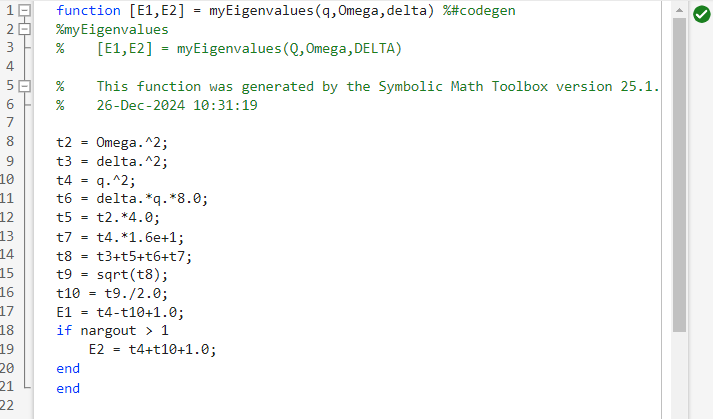• The Code Analyzer message indicator in the top right corner of the MATLAB Editor is green. The analyzer did not detect errors, warnings, or opportunities for improvement in the code. For more information about using the Code Analyzer, see Check Code for Errors and Warnings Using the Code Analyzer.

• Save the file. You are now ready to compile your code by using the MATLAB Coder app. Here, compilation refers to the generation of C/C++ code from your MATLAB code.

#### Open MATLAB Coder App and Select Source Files

• On the MATLAB toolstrip Apps tab, under Code Generation, click the MATLAB Coder app icon. The app opens the Select Source Files page.

• In the Select Source Files page, enter or select the name of the entry-point function `myEigenvalues`. An entry-point function is a top-level MATLAB function from which you generate code. The app creates a project with the default name `myEigenvalues.prj` in the current folder.• Click Next to go to the Define Input Types step. The app runs the Code Analyzer, which you already ran in the previous step, and the Code Generation Readiness Tool on the entry-point function. If the app identifies issues, it opens the Review Code Generation Readiness page where you can review and fix issues. In this example, because the app does not detect issues, it opens the Define Input Types page. For more information, see Code Generation Readiness Tool (MATLAB Coder).

Note that the Code Analyzer and the Code Generation Readiness Tool might not detect all code generation issues. After eliminating the errors or warnings that these two tools detect, generate code with MATLAB Coder to determine if your MATLAB code has other compliance issues.

Certain MATLAB built-in functions and toolbox functions, classes, and System objects that are supported for C/C++ code generation have specific code generation limitations. These limitations and related usage notes are listed in the Extended Capabilities sections of their corresponding reference pages. For more information, see Functions and Objects Supported for C/C++ Code Generation (MATLAB Coder).

#### Define Input Types

Because C uses static typing, the code generator must determine the class, size, and complexity of all variables in the MATLAB files at code generation time, also known as compile time. Therefore, you must specify the properties of all entry-point function inputs. To specify input properties, you can:

• Instruct the app to automatically determine input properties by providing a script that calls the entry-point functions with sample inputs.

• Specify properties directly.

In this example, to define the properties of the inputs `q`, `delta`, and `Omega`, specify the test file `myTest.m` for the code generator to use to define types automatically:

• Enter or select the test file `myTest.m` in the MATLAB prompt.

• Click Autodefine Input Types.The test file, `myTest.m`, calls the entry-point function, `myEigenvalues`, with the expected input types. The app determines that the input `q` is `double(128 x 256)`, the input `Omega` is `double(128 x 256)`, and the input `delta` is `double(1 x 1)`.• Click Next to go to the Check for Run-Time Issues step.

#### Check for Run-Time Issues

The Check for Run-Time Issues step generates a MEX function from your entry-point functions, runs the MEX function, and reports issues. A MEX function is generated code that can be called from inside MATLAB. Performing this step is a best practice because you can detect and fix run-time errors that are harder to diagnose in the generated C code. By default, the MEX function includes memory integrity checks. These checks perform array bounds and dimension checking. The checks detect violations of memory integrity in code generated for MATLAB functions. For more information, see Control Run-Time Checks (MATLAB Coder).

To convert MATLAB code to efficient C/C++ source code, the code generator introduces optimizations that, in certain situations, cause the generated code to behave differently than the original source code. See Differences Between Generated Code and MATLAB Code (MATLAB Coder).

• To open the Check for Run-Time Issues dialog box (if the dialog box does not automatically appear), click the Check for Issues arrow.

• In the Check for Run-Time Issues dialog box, specify a test file or enter code that calls the entry-point function with example inputs. For this example, use the test file `myTest` that you used to define the input types.

• Click Check for Issues. The app generates a MEX function that can be run inside MATLAB. This step runs the test script `myTest` replacing calls to `myEigenvalues` with calls to the generated MEX function, that is `[E1,E2] = myEigenvalues_mex(qGrid,OmegaGrid,delta)`. The generated MEX file `myEigenvalues_mex` is located in the folder `work``\codegen\lib\myEigenvalues` (on Microsoft® Windows® platforms) or `work``/codegen/lib/myEigenvalues` (on Linux® or Mac platforms), where `work` is the location of `myEigenvalues.m` and `myTest.m`. If the app detects issues during the MEX function generation or execution, it provides warning and error messages. Click these messages to navigate to the problematic code and fix the issue. In this example, the app does not detect issues.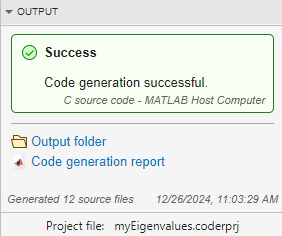• By default, the app collects line execution counts. These counts help you see how well the test file `myTest.m` exercised the `myEigenvalues` function. To view line execution counts, click View MATLAB line execution counts. The app editor displays a color-coded bar to the left of the code. To extend the color highlighting over the code and to see line execution counts, place your cursor over the bar. A particular shade of green indicates that the code only executes one call to compute the eigenvalues.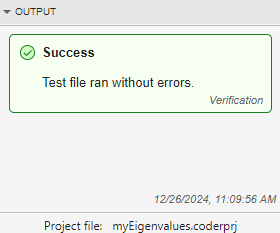• Click Next to go to the Generate Code step.

#### Generate C Code

• To open the Generate dialog box (if the dialog box does not automatically appear), click the Generate arrow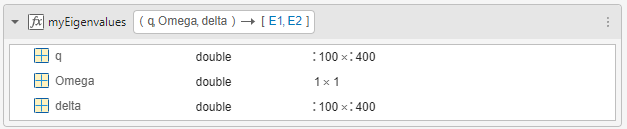.

• In the Generate dialog box, set Build type to `Static Library(.lib)` and Language to C. Use the default values for the other project build configuration settings. Instead of generating a C static library, you can choose to generate a MEX function or other C/C++ build types. Different project settings are available for the MEX and C/C++ build types. When you switch between MEX and C/C++ code generation, verify the settings that you choose.• Click Generate. MATLAB Coder generates a standalone C static library, `myEigenvalues`, in the folder `work``\codegen\lib\myEigenvalues`. The folder `work` is the location of `myEigenvalues.m` and `myTest.m`. The MATLAB Coder app indicates when code generation has succeeded. It displays the source MATLAB files and generated output files on the left side of the page. On the Variables tab, it displays information about the MATLAB source variables. On the Target Build Log tab, it displays the build log, including C/C++ compiler warnings and errors. By default, the code window displays the C source code file, `myEigenvalues.c`. To view a different file, click the desired file name in the Source Code or Output Files pane.• Click View Report to view the report in the Report Viewer. If the code generator detects errors or warnings during code generation, the report describes the issues and provides links to the problematic MATLAB code. For more information, see Code Generation Reports (MATLAB Coder).

• Click Next to open the Finish Workflow page.

#### Review Finish Workflow Page

The Finish Workflow page indicates that code generation has succeeded. It provides a project summary and links to generated output.#### Compare Generated C Code to OriginalMATLABCode

To compare your generated C code to the original MATLAB code, open the C file, `myEigenvalues.c`, and the `myEigenvalues.m` file in the MATLAB Editor.

Important information about the generated C code:

• The function signature is:

```void myEigenvalues(const double q, const double Omega, double delta, double E1, double E2) ```
• `const double q` and `const double Omega` corresponds to the input `q` and `Omega` in your MATLAB code. The size of `q` is `32768`, which corresponds to the total size `(128 x 256)` of the example input that you used when you generated C/C++ code from your MATLAB code. The same applies to the input `Omega`. In this case, the generated code uses one-dimensional arrays to represent two-dimensional arrays in the MATLAB code.

• The code generator preserves your function name and comments. When possible, the code generator preserves your variable names. Note that if a variable in your MATLAB code is set to a constant value, it does not appear as a variable in the generated C code. Instead, the generated C code contains the value of the variable as a literal.

### Generate C Code for Variable-Size Inputs

The C function that you generated for `myEigenvalues.m` can accept only inputs that have the same size as the sample inputs that you specified during code generation. However, the input arrays to the corresponding MATLAB function can be of any size. In this part of the example, you generate C code from `myEigenvalues.m` that accepts variable-size inputs.

Suppose that you want the dimensions of `q`, `Omega`, and `delta` in the generated C code to have these properties:

• The first dimension of both `q` and `delta` can vary in size up to `100`.

• The second dimension of `q` and `delta` can vary in size up to `400`.

• `Omega` is a scalar of size `1`-by-`1`.

To specify these input properties using MATLAB Coder, follow these steps:

• In the Define Input Types step, specify the test file `myTest.m` and click Autodefine Input Types as before. The test file calls the entry-point function, `myEigenvalues.m`, with the expected input types. The app determines that the input `q` is `double(128 x 256)`, the input `Omega` is `double(128 x 256)`, and the input `delta` is `double(1 x 1)`. These types specify fixed-size inputs.

• Click the input type specifications to edit them. You can specify variable size, up to a specified limit, by using the `:` prefix. For example, `:100` specifies that the corresponding dimension can vary in size up to `100`. Change the type for `q` to `double(:100 x :400)`, for `Omega` to `double(1 x 1)`, and for `delta` to `double(:100 x :400)`.You can now generate code by following the same steps as before. The function signature for the generated C code in `myEigenvalues.c` now reads:

```void myEigenvalues(const emxArray_real_T *q, double Omega, const emxArray_real_T *delta, emxArray_real_T *E1, emxArray_real_T *E2) ```

The arguments in the generated code correspond to these arguments in the original MATLAB function:

• `emxArray_real_T*q` — the `q` input argument

• `Omega` — the `Omega` input argument

• `emxArray_real_T*delta` — the `delta` input argument

• `emxArray_real_T*E1` — the `E1` output argument

• `emxArray_real_T*E2` — the `E2` output argument

The C code now consists of a data structure called an `emxArray_real_T` to represent an array whose size is unknown and unbounded at compile time. For more details, see Use C Arrays in the Generated Function Interfaces (MATLAB Coder).

## Support

#### Mathematical Modeling with Symbolic Math Toolbox

Get examples and videos